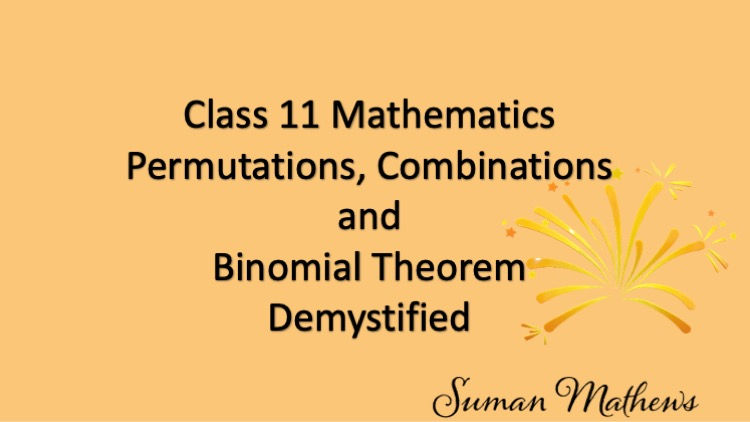top of page
Search

# Class 11 Mathematics-Permutations, Combinations and Binomial Theorem Demystified

Updated: Nov 8## Permutations, Combinations and Binomial Theorem

Once upon a time, in a quiet town there was a bakery owned by a kind hearted baker, named Mr. White. He had three types of delicious pastries, chocolate, strawberry and vanilla. As part of his sales, he had a special box of pastries where a customer could choose how many pastries to include and which pastries.

There were a few conditions:

1. The customer could choose two to four pastries.

2. He could select any combination of flavors including duplicates.

3. The order of the pastries did not matter.

How many different combinations could the customer choose? Suppose the customer chooses two pastries. Since there were three types and repetition was allowed, the customer had three choices for the first and three choices for the second.

Hence, the customer had 9 possibilities. Likewise, if the customer chose three pastries, then there were twenty seven possibilities . For a box of four pastries, a customer would have 81 possibilities.

So the customer had 9+27+81=117 possibilities since he could choose two or three or four pastries. Note that when it is "OR' we add. This is how, Permutations and Combinations can make a seemingly complex mathematical concept come to life.

Welcome to Permutations and Combinations. Permutations and Combinations form an integral part of Algebra and is also used extensively in Probability. I will be giving you an overview of this chapter and the important topics that you should be knowing.

A good knowledge of Permutations and Combinations helps in answering SAT subject level exams and the GRE QUANT exams. Each problem has a different approach here and there is no standard formula applying to every problem.

You can contact me for online classes if you need further help. To start with you will need to understand the factorial notation and how to solve problems using factorial. Next, you'll need to learn about the Fundamental Principle of Counting.

### ​Permutation as an Arrangement

This is basically the principal concept used in every problem in this chapter. A permutation is basically an arrangement of objects. The number of permutations of n different objects, taken r at a time is denoted by P(n,r).

You start with the formula for permutations and use it in problem solving. An interesting case is when you have to find the number of permutations when two or more objects are together. In this case, you take these as one object and find the number of permutations of the remaining objects. Finally you have to remember to permute these objects also.

The next important point is to find the number of permutations when all the objects are not different. That is, you find the number of permutations of n objects taken all together, when p objects are alike of one kind, q of them are alike of another kind and r of them are alike of a third kind. These type of problems are used while considering the arrangements of alphabets.

Permutations and Combinations-Part two

#### Circular Permutations​

You'll then proceed to learn about Circular Permutations. The number of permutations of n objects around a circular table is (n-1)! The number of ways of arranging n persons around a round table so that no person has the same two neighbours is (n-1)!/2. This is because the clockwise and anticlockwise arrangements are not different.

Cyclic Permutations explained​

##### ​What are Combinations?

Moving on to Combinations, keep in mind that a combination is a selection. Learn how to find the combinations of n different objects taken r at a time. Again, there are a number of properties in Combinations which you'll be learning.

You'll also learn how to solve mixed problems which have both Permutations and Combinations. These problems are slightly tricky requiring an in depth understanding. Feel free to join my online classes for Permutations and Combinations.

You'll learn formulas, Concepts and problem solving skills.

Formulas on Permutations and Combinations

###### ​Binomial Theorem

Coming to Binomial Theorem, you need to have some knowledge of Combinations, before starting this chapter. Basically, the Binomial Theorem helps you to expand powers of (a+b).The coefficients of the powers of a and b are called Binomial coefficients.

Note, that the formula to evaluate powers of (a-b) is the same as a+b, only we replace b by -b. You can use this theorem to find the number of terms of (a+b)^n. You'll then learn the formula to find the general term of (a+b)^n.

So that's permutations, combinations and Binomial theorem demystified.

This formula also helps you to determine the middle term of (a+b)^n. the constant term or the coefficient of any power of a or b. This is a fairly easy topic. However, if you need further help, you can join my online classes.